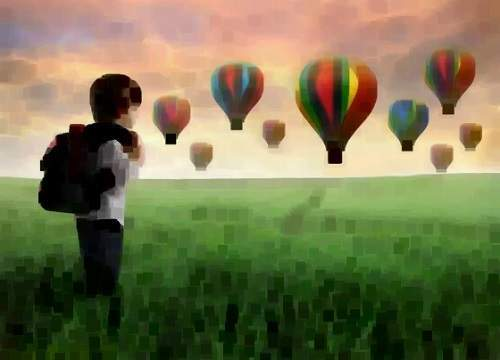# Java DIP - Eroding and Dilating

In this chapter we learn apply two very common morphology operators:Dilation and Erosion.

We use OpenCV function erode and dilate. They can be found under Imgproc package. Its syntax is given below −

```Imgproc.erode(source, destination, element);
Imgproc.dilate(source, destination, element);
```

The parameters are described below −

Sr.No. Parameter & Description
1

source

It is Source image.

2

destination

It is destination image.

3

element

It is a structuring element used for erosion and dilation, if element=Mat(), a 3 x 3 rectangular structuring element is used.

Apart from erode() and dilate() methods, there are other methods provided by the Imgproc class. They are described briefly −

Sr.No. Method & Description
1

cvtColor(Mat src, Mat dst, int code, int dstCn)

It converts an image from one color space to another.

2

dilate(Mat src, Mat dst, Mat kernel)

It dilates an image by using a specific structuring element.

3

equalizeHist(Mat src, Mat dst)

It equalizes the histogram of a grayscale image.

4

filter2D(Mat src, Mat dst, int depth, Mat kernel, Point anchor, double delta)

It convolves an image with the kernel.

5

GaussianBlur(Mat src, Mat dst, Size ksize, double sigmaX)

It blurs an image using a Gaussian filter.

6

integral(Mat src, Mat sum)

It calculates the integral of an image.

## Example

The following example demonstrates the use of Imgproc class to perform erosion and dilation on an image −

```import org.opencv.core.Core;
import org.opencv.core.CvType;
import org.opencv.core.Mat;
import org.opencv.highgui.Highgui;
import org.opencv.imgproc.Imgproc;

public class main {
public static void main( String[] args ) {

try{
Mat destination = new Mat(source.rows(),source.cols(),source.type());

destination = source;

int erosion_size = 5;
int dilation_size = 5;

Mat element = Imgproc.getStructuringElement(Imgproc.MORPH_RECT, new  Size(2*erosion_size + 1, 2*erosion_size+1));
Imgproc.erode(source, destination, element);
Highgui.imwrite("erosion.jpg", destination);

destination = source;

Mat element1 = Imgproc.getStructuringElement(Imgproc.MORPH_RECT, new  Size(2*dilation_size + 1, 2*dilation_size+1));
Imgproc.dilate(source, destination, element1);
Highgui.imwrite("dilation.jpg", destination);

} catch (Exception e) {
System.out.println("error:" + e.getMessage());
}
}
}
```

## Output

When you execute the given code, the following output is seen −

### Original ImageOn the above original image, some erosion and dilation operations have been performed which have been shown in the output below −

### Erosion### Dilation